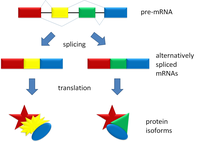# Introduction to Alternative Splicing solved by 1730

Dec. 4, 2012, 7:05 a.m. by Rosalind Team

Topics: Combinatorics

## The Baby and the BathwaterFigure 1. Alternative splicing induces different protein isoforms.

In “RNA Splicing”, we described the process by which the exons are spliced out from a molecule of pre-mRNA and reassembled to yield a final mRNA for the purposes of protein translation.

However, the chaining of exons does not always proceed in the same manner; alternative splicing describes the fact that all the exons from a gene are not necessarily joined together in order to produce an mRNA. The most common form of alternative splicing is exon skipping, in which certain exons are omitted along with introns.

Alternative splicing serves a vital evolutionary purpose, as it greatly increases the number of different proteins that can be translated from a given gene; different proteins produced from the same gene as a result of alternative splicing are called protein isoforms; see Figure 1 In fact, about 95% of human genes are commonly spliced in more than one way. At the same time, when alternative splicing goes wrong, it can create the same negative effects caused by mutations, and it has been blamed for a number of genetic disorders.

In this problem, we will consider a simplified model of alternative splicing in which any of a collection of exons can be chained together to create a final molecule of mRNA, under the condition that we use a minimum number of exons ($m$) whose order is fixed. Because the exons are not allowed to move around, we need only select a subset of at least $m$ of our exons to chain into an mRNA.

The implied computational question is to count the total number of such subsets, which will provide us with the total possible number of alternatively spliced isoforms for this model.

## Problem

In “Counting Subsets”, we saw that the total number of subsets of a set $S$ containing $n$ elements is equal to $2^n$.

However, if we intend to count the total number of subsets of $S$ having a fixed size $k$, then we use the combination statistic $C(n, k)$, also written $\binom{n}{k}$.

Given: Positive integers $n$ and $m$ with $0 \leq m \leq n \leq 2000$.

Return: The sum of combinations $C(n, k)$ for all $k$ satisfying $m \leq k \leq n$, modulo 1,000,000. In shorthand, $\sum_{k=m}^{n}{\binom{n}{k}}$.

## Sample Dataset

6 3


## Sample Output

42